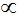## Thursday, 9 July 2015

### Ideal Gas Equation

Combination of 3 laws (Boyles Law, Charles Law and Avogadro law) gives a single equation (PV=nRT) called as Ideal gas equation.
Mathematically,
According to Boyle’s Law; at constant T and n,
V1/P ……….1
According to Charles Law; at constant P and n,
VT ……….2
According to Avogadro Law; at constant T and n,
Vn  ……….3
From equation 1, 2 and 3; we get,
VnT/P ……….4
Or, V =R nT/P ……….5
Also, PV = nRT  …………..6
Then, R = PV/nT  ………..7
Where, R is a gas constant which is same for all gases and known as Universal Gas Constant and equation 6, PV = nRT is known as Ideal Gas Equation.

## Equation Of State

Ideal gas equation is also known as equation of state because it gives relationship between 4 variables i.e. P, V, n and T. which describes state of any gas.
Let  if pressure, volume and temperature of fixed amount of ideal gas changes from P1, V1, T1 to P2, V2, T2  then,
P1V1/T1 = nR …………..8
P2V2/T2 = nR …………..9
So, from equation 8 & 9, we get
P1V1/T1 = P1V1/T1 ………..10
This above equation (eq. 10) is called Combined Gas Law.

#### Density And Molar Mass Of Gaseous Substances:

As per Ideal Gas Equation,
PV=nRT
Then, n/V = P/RT
On replacing n by m/M (as mole n= mass m/ molar mass M); we obtain,
m/MV=P/RT
On replacing m/V by density d; we obtain,
d/M=P/RT
Also, on rearrangement,
M = dRT/P
Where, M is molar mass, d is density, R is gas constant, T is temperature and P is pressure.

### Dalton Law Of Partial Pressure:

According to Dalton law of partial pressure, “Total exerted pressure by mixture of all non-reactive gases is equal to the sum of partial pressure of all individual gases.”
At constant temperature T and Volume V
Ptotal = p1 +p2 +p3…………..
Where, Ptolal = total exerted pressure of mixture of all gases.
p1, p2, p3 etc. is pressure exerted by individual gases known as partial pressure.

#### Aqueous Tension:

It is exerted by the saturated water vapors.
Pdrygas = Ptotal – Aqueous Tension

#### Partial Pressure In Terms Of Mole Fraction:

Let at T temperature, 3 gases of Volume V exert the partial pressure p1, p2, p3. Then as per ideal gas equation,
p1=n1RT/V
p2=n2RT/V
p3=n3RT/V
Where, n1, n2, n3 are no. of moles.
Also, according to Daltons law of partial pressure
Ptotal = p1 +p2 +p3
Or, Ptotal = n1RT/V + n2RT/V + n3RT/V = (n1+n2+n3)RT/V
And, on dividing p1 by PTotal , we obtain
P1/ PTotal={n1/(n1+n2+n3)}{RTV/RTV}
P1/ PTotal=n1/(n1+n2+n3) = n1/n = x1
Where, n= n1+n2+n3 and x1 is mole fraction of first gas.
So, p1=x1PTotal
Similarly, p2=x2PTotal
P3=x3PTotal
Then, general equation is written as-
Pi=xiPTotal
Where, pi is partial pressure of ith gas.
xi is mole fraction of ith gas.